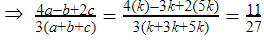# Previous Year Ratio and Proportion Questions for SSC CHSL 2021

Validity: 9 Months
What you will get
Course Highlights
• Based on latest Pattern
• English Medium eBooks
SSC

## Previous Year Ratio and Proportion Questions for SSC CHSL 2021

In this article, we provide previous year questions (date-wise) of SSC CHSL 2019 asked by TCS  in Hindi and English. These questions are the most important for upcoming exams of SSC CGL, CHSL, CPO, and various others.

Q1. A sum of Rs. 4360 was to be divided among A, B, C, and D in the ratio of 3 : 4 : 5 : 8, but it was divided in the ratio of 1/3:1/4:1/5:1/8 by mistake. As a result:

4360 रुपये की एक राशि को A, B, C तथा D के बीच 3 : 4 : 5 : 8 के अनुपात में विभाजित करना था, लेकिन इसे भूलवश  1/3:1/4:1/5:1/8 के अनुपात में विभाजित कर दिया गया | परिणामस्वरूप :

SSC CHSL 1 July 2019 (Evening)

(a) A received Rs. 956 more/ A को 956 रुपये अधिक मिले |

(b) B received Rs. 318 more/ B को 318 रुपये अधिक मिले |

(c) D received Rs. 1144 less/ D को 1144 रुपये कम मिले |

(d) C received Rs. 132 less/ C को 132 रुपये कम मिले |

Q2.Two numbers are in the ratio 3:4. On increasing each of them by 30, the ratio becomes 9:10. The numbers are:

दो संख्याएं 3 : 4 के अनुपात में हैं | दोनों को 30 से बढ़ाने पर, यह अनुपात 9 : 10 हो जाता है | ये संख्याएं हैं :

SSC CHSL 8 July 2019 (Afternoon)

(a) 30,40

(b) 15,20

(c) 12,16

(d) 18,24

Q3. The ratio of incomes of A and B is 2 : 3 and that of their expenditure is 1 : 2. If 90% of B’s expenditure is equal to the income of A, then what is the ratio of the savings of A and B?

A और B की आय का अनुपात 2 : 3 है तथा उनके व्यय का अनुपात 1 : 2 है | यदि B का 90% व्यय A की आय के बराबर है, तो A और B की बचत का अनुपात ज्ञात करें |

SSC CHSL 2 July 2019 (Morning)

(a) 1 : 1

(b) 9 : 8

(c) 8 : 7

(d) 3 : 2

Q4. In an examination, the success to failure ratio was 5 : 2. Had the number of failures been 14 more, then the success to failure ratio would have been 9 : 5. The total number of candidates who appeared for the examination was :

एक परीक्षा में, सफलता और असफलता का अनुपात 5 : 2 था | यदि असफल अभ्यर्थी की संख्या  14 अधिक होती , तो सफलता और असफलता का अनुपात 9 : 5 होता | इस परीक्षा में कुल कितने अभ्यर्थी शामिल हुए थे ?

SSC CHSL 2 July 2019 (Evening)

(a) 210

(b) 196

(c) 126

(d) 203

Q5. Three numbers are in the ratio  ½ : ⅔ : ¾ . The difference between the greatest and the smallest number is 27. The smallest number is

तीन संख्याएं :  ½ : ⅔ : ¾  के अनुपात में हैं | सबसे बड़ी तथा सबसे छोटी संख्याओं के बीच 27 का अंतर है | सबसे छोटी संख्या है -

SSC CHSL 4 July 2019 (Evening)

(a) 81

(b) 40

(c) 72

(d) 54

Q6. Three numbers are in the ratio  ½ : ⅔ : ¾ . The difference between the greatest and the smallest number is 27. The average of the three numbers is :

तीन संख्याएं ½ : ⅔ : ¾ के अनुपात में हैं | सबसे बड़ी तथा सबसे छोटी संख्या के बीच 27 का अंतर है | तीनों संख्याओं का औसत ज्ञात करें |

SSC CHSL 5 July 2019 (Morning)

(a) 40

(b) 81

(c) 69

(d) 54

Q7. If a:b:c=1:3:5, what is the value of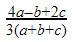यदि a:b:c=1:3:5 है, तोका मान क्या होगा ?

SSC CHSL 8 July 2019 (Evening)

(a) 827

(b) 1027

(c) 1127

(d) 13

Q8. Rs. 8000 is distributed among A, B and C such that they receive notes of Rs. 500, Rs. 200 and Rs. 100 respectively. The amounts received by them are in the ratio 15:2:3. What was the ratio of the numbers of notes of Rs. 500, Rs 200 and Rs 100 ? 8000 रुपये A, B और C के बीच इस प्रकार वितरित किये जाते हैं कि उन्हें क्रमशः 500, 200 और 100 रुपये के नोट प्राप्त होते हैं | उनके द्वारा प्राप्त की गयी राशि का अनुपात 15 : 2 : 3 है | 500, 200 तथा 100 रुपये के नोटों की संख्या का अनुपात ज्ञात करें |

SSC CHSL 10 July 2019 (Morning)

(a) 3:1:3

(b) 3:3:1

(c) 4:1:2

(d) 3:2:2

Q9. What is the ratio between the fourth proportional of 3, 4, 9 and the mean proportional between 2 and 98?

3, 4, 9 के चतुर्थ अनुपाती और 2 और 98 के मध्यानुपाती के बीच का अनुपात क्या है?

SSC CHSL 11 July 2019 (Afternoon)

(a) 7:8

(b) 7:6

(c) 8:7

(d) 6:7

Q10. The ratio of the present ages of A and B is 6 : 5. Four years ago, this ratio was 5 : 4. What will be the ratio of ages of A and B after 12 years from now?

A और B की वर्तमान आयु का अनुपात 6 : 5 है | चार वर्ष पहले, यह अनुपात 5 : 4 था | अब से 12 वर्ष बाद A और B की उम्र में क्या अनुपात होगा ?

SSC CHSL 2 July 2019 (Afternoon)

(a) 3 : 2

(b) 8 : 7

(c) 9 : 8

(d) 7 : 6

Q11. Eight years ago, the ratio of ages of A and B was 9 : 10. The ratio of their ages four years from now will be 12 : 13. What is the age (In years) of C now, if his age is 6 years more than that of A?

आठ वर्ष पहले, A और B की उम्र में 9 : 10 का अनुपात था | अब से चार वर्ष बाद उनकी उम्र का अनुपात 12: 13 हो जाएगा | C की वर्तमान आयु ( वर्ष में ) ज्ञात करें, यदि उसकी उम्र A की उम्र से 6 साल अधिक है |

SSC CHSL 3 July 2019 (Morning)

(a) 56

(b) 42

(c) 50

(d) 48

Q12. Two numbers A and B are in the ratio 5 : 2. If 4 is added to each number then this ratio becomes 9 : 4. If 5 is subtracted from each of the original numbers , then the ratio of A and B will be:

दो संख्याएं A और B 5 : 2 के अनुपात में हैं | यदि प्रत्येक संख्या में 4 जोड़ा जाए, तो यह अनुपात 9 : 4 हो जाता है | यदि प्रत्येक मूल संख्या में से 5 घटाया जाए, तो A और B का अनुपात क्या हो जाएगा ? SSC CHSL 3 July 2019 (Afternoon)

(a) 3 : 1

(b) 8 : 3

(c) 7 : 2

(d) 4 : 1

Q13. Incomes of A and B are in the ratio 5:3 and their expenditure are in the ratio 9:5. If income of A is twice the expenditure of B, then what is the ratio of savings of A and B?

A और B की आय 5 : 3 के अनुपात में है  और उनके व्यय 9 : 5 के अनुपात में हैं | यदि A की आय B के व्यय से दोगुनी है, तो A और B की बचत का अनुपात ज्ञात करें |

SSC CHSL 4 July 2019 (Morning)

(a) 2:3

(b) 1:1

(c) 3:2

(d) 3:4

Q14. Two numbers are in the ratio 7:5. On diminishing each of them by 40, the ratio becomes 27:17. The difference between the numbers is :

दो संख्याएं 7 : 5 के अनुपात में हैं | दोनों में से 40 घटाने पर, यह अनुपात 27 : 17 हो जाता है | इन दोनों संख्याओं के बीच क्या अंतर है ?

SSC CHSL 5 July 2019 (Afternoon)

(a) 75

(b) 40

(c) 25

(d) 50

Q15. Two numbers are in the ratio 7:5. On diminishing each of them by 40, the ratio becomes 27:17. The sum of the numbers is :

दो संख्याएं 7 : 5 के अनुपात में हैं | दोनों में से 40 घटाने पर, यह अनुपात 27 : 17 हो जाता है | इन दोनों संख्याओं का योग ज्ञात करें |

SSC CHSL 5 July 2019 (Evening)

(a) 300

(b) 240

(c) 325

(d) 275

Q16. Two numbers are in the ratio 3:4. On increasing each of them by 30, the ratio becomes 9:10. The sum of the numbers is :

दो संख्याएं 3 : 4 के अनुपात में है | दोनों को 30 से बढ़ाने पर, यह अनुपात 9 : 10 हो जाता है | इन संख्याओं का योग है - SSC CHSL 8 July 2019 (Morning)

(a) 35

(b) 32

(c) 30

(d) 25

Q17.  What is the ratio of mean proportional of 14.4 and 3.6 and the third proportional of 5 and 4?

14.4 और 3.6 के बीच माध्य समानुपाती और 5 तथा 4 के तीसरे आनुपातिक का अनुपात क्या है ?

SSC CHSL 11 July 2019 (Evening)

(a) 4:9

(b) 5:8

(c) 9:4

(d) 8:5

Q18. If a:b:c = 1:3:5, what is the value of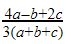?

यदि a:b:c = 1:3:5 है, तोका मान क्या होगा ?

SSC CHSL 11 July 2019 (Evening)

(a) 827

(b) 1027

(c) 1127

(d) 13

Here are the Solutions to the above questions of Ratio and Proportion asked by TCS in SSC CHSL exam.

Sol 1. (c)

Actual ratio = 3:4:5:8

⇒ (3+4+5+8) unit = 4360

⇒ 20 unit = 4360

⇒ 1 unit = 218

A’s share (3 unit) = 3 ×218 = 654

B’s share (4 unit) = 4 ×218 = 872

C’s share (5 unit) = 5 ×218 = 1090

D’s share (8 unit) = 8 ×218 = 1744

Mistaken ratio = 1/3:1/4:1/5:1/8=40:30:24:15

⇒ (40+30+24+15) unit = 4360

⇒ 1 unit = 40

A’s share (40 unit) = 40 × 40 = 1600

B’s share (30 unit) = 30 × 40 = 1200

C’s share (24 unit) = 24 × 40 = 960

D’s share (15 unit) = 15 × 40 = 600

Clearly D get 1744-600 =  Rs.1144 less

Sol 2. (b)

Let the numbers are 3k and 4k

According to the question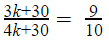⇒ 30k+300 = 36k+270

⇒ 6k=30

⇒ k=5

So, the numbers are 3 ×5= 15 and 4 ×5= 20 ans

Sol 3. (c)

Let, the incomes of A and B  are 2I and 3I respectively.

The expenditure of A and B are 1E and 2E respectively.

According to the question

2I = 2E  × 90/100

IE= 910

Saving of A = 2(9)-1(10) = 8 unit

Saving of B = 3(9)-2(10) = 7 unit

Required ratio = 8 : 7 ans

Sol 4. (b)

Let the number of successful candidates and failure candidates 5k and 2k.

According to the question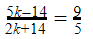⇒ 25k - 70 = 18k + 126

⇒ k = 28

Total number of students = 5k+2k = 7k

= 728 = 196

Sol 5.(d)

½ : ⅔ : ¾ ⇒ 6:8:9

Let the numbers 6k , 8k and 9k

According to the question

9k-6k = 27

⇒k = 9

Smallest number (6k)= 6 × 9 = 54

Sol 6. (c)

½ : ⅔ : ¾ 6:8:9

Let the numbers 6k , 8k and 9k

According to the question

9k-6k = 27

k = 9

Average of three numbers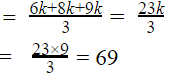Sol 7. (c)

Given, a:b:c=1:3:5

Let a = k, b=3k and c = 5k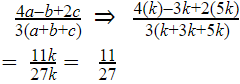Sol 8.(a)

Ratio of amount received = 15:2:3

Ratio of note’s worth = 500 : 200: 100

= 5 : 2 : 1

Number of notes = Total amount/note's worth

Ratio of number of notes = 15/5:2/2:3/1= 3:1:3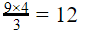Sol 9. (d)

fourth proportional of 3, 4, 9 =mean proportional between 2 and 98 =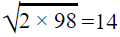Required ratio = 12 : 14

= 6 : 7

Sol 10. (c)

Let the age of A and B be 6k and 5k respectively.

According to the question⇒ 24k-16 = 25k-20

⇒ k=4

Required ratio = 6(4)+12 : 5(4)+12

= 9 : 8

Sol 11. (c)

Let the ages of A and B 8 years ago were 9k and 10k respectively.

According to the question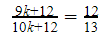⇒ 17k+156 = 120k+144

⇒ k=4

Present age of A = 9(4)+8 = 44 years

Present age of C = 44+6 = 50 years

Sol 12. (a)

Let the value of A and B be 5k and 2k respectively.

According to the question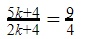⇒ 20k+16 = 18k+36

⇒ k=10

Required ratio = 5(10)-5 : 2(10)-5

= 3:1

Sol 13. (b)

Let the income of A and B be 5I and 3I respectively.

Let the expenditure of A and B be 9E and 5E.

According to the question

5I = 2(5E)

I/E= 2/1

Savings of A = 5(2)-9(1) = 1 unit

Savings of B = 3(2)-5(1) = 1unit

Required ratio = 1:1

Sol 14. (d)

Let the numbers are 7k and 5k.

Difference of the numbers = 7k-5k = 2k

According to the question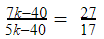119k-680 = 135k - 1080

k=25

Required difference = 2(25) = 50

Sol 15. (a)

Let the numbers are 7k and 5k.

Sum of the numbers = 7k+5k = 12k

According to the question⇒119k-680 = 135k - 1080

⇒k=25

Required sum = 12(25) = 300

Sol 16. (a)

Let the numbers are 3k and 4k.

Sum of the numbers = 3k+4k = 7k

According to the question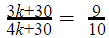⇒30k+300 = 36k + 270

⇒k=5

Required sum = 7(5) = 35

Sol 17. (c)

third proportional of 5, 4 =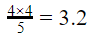mean proportional between 14.4 and 3.6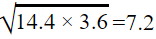Required ratio = 7.2 : 3.2

= 9 : 4

Sol 127. (c)

Let a = k, b = 3k and c = 5k